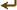[frames] | no frames]

# Package impexpd

source code

Classes and functions for import/export daemon.

 Classes
CommandBuilder
ChildIOProcessor
 Functions

float or None
 _CalcThroughput(samples) Calculates the throughput in MiB/second. source code
 Variables
LISTENING_RE = `re.compile(r"^listening on\s+" r"AF=(?P<family>...`
Used to recognize point at which socat(1) starts to listen on its socket.
TRANSFER_LOOP_RE = `re.compile(r"^starting data transfer loop w...`
Used to recognize point at which socat(1) is sending data over the wire
SOCAT_LOG_DEBUG = `"D"`
SOCAT_LOG_INFO = `"I"`
SOCAT_LOG_NOTICE = `"N"`
SOCAT_LOG_WARNING = `"W"`
SOCAT_LOG_ERROR = `"E"`
SOCAT_LOG_FATAL = `"F"`
SOCAT_LOG_IGNORE = `compat.UniqueFrozenset([SOCAT_LOG_DEBUG, SO...`
DD_INFO_RE = `re.compile(r"^(?P<bytes>\d+)\s*byte(?:|s)\s.*\sco...`
Used to parse GNU dd(1) statistics
DD_STDERR_IGNORE = `re.compile(r"^\d+\+\d+\s*records\s+(?:in|ou...`
Used to ignore "N+N records in/out" on dd(1)'s stderr
DD_INFO_SIGNAL = `getattr(signal, "SIGINFO", signal.SIGUSR1)`
Signal upon which dd(1) will print statistics (on some platforms, SIGINFO is unavailable and SIGUSR1 is used instead)
BUFSIZE = `1024* 1024`
Buffer size: at most this many bytes are transferred at once
SOCAT_TCP_OPTS = `["keepalive", "keepidle=60", "keepintvl=10", ...`
SOCAT_OPENSSL_OPTS = `["verify=1", "method=TLSv1", "cipher=%s" ...`
SOCAT_OPTION_MAXLEN = `400`
PROG_ALL = `compat.UniqueFrozenset([PROG_OTHER, PROG_SOCAT, PRO...`

Imports: os, re, socket, logging, signal, errno, time, StringIO, constants, errors, utils, netutils, compat

 Function Details

### _CalcThroughput(samples)

source code

Calculates the throughput in MiB/second.

Parameters:
• `samples` (sequence) - List of samples, each consisting of a (timestamp, mbytes) tuple
Returns: float or None
Throughput in MiB/second

 Variables Details

### LISTENING_RE

Used to recognize point at which socat(1) starts to listen on its socket. The local address is required for the remote peer to connect (in particular the port number).

Value:
 ```re.compile(r"^listening on\s+" r"AF=(?P\d+)\s+" r"(?P.+):(?P\d+)\$", re.I) ```

### TRANSFER_LOOP_RE

Used to recognize point at which socat(1) is sending data over the wire

Value:
 ```re.compile(r"^starting data transfer loop with FDs\s+.*\$", re.I) ```

### SOCAT_LOG_IGNORE

Value:
 ```compat.UniqueFrozenset([SOCAT_LOG_DEBUG, SOCAT_LOG_INFO, SOCAT_LOG_NOTICE,]) ```

### DD_INFO_RE

Used to parse GNU dd(1) statistics

Value:
 ```re.compile(r"^(?P\d+)\s*byte(?:|s)\s.*\scopied,\s*" r"(?P[\d.]+)\s*s(?:|econds),.*\$", re.I) ```

### DD_STDERR_IGNORE

Used to ignore "N+N records in/out" on dd(1)'s stderr

Value:
 ```re.compile(r"^\d+\+\d+\s*records\s+(?:in|out)\$", re.I) ```

### SOCAT_TCP_OPTS

Value:
 ```["keepalive", "keepidle=60", "keepintvl=10", "keepcnt=5"] ```

### SOCAT_OPENSSL_OPTS

Value:
 ```["verify=1", "method=TLSv1", "cipher=%s" % constants.OPENSSL_CIPHERS] ```

### PROG_ALL

Value:
 ```compat.UniqueFrozenset([PROG_OTHER, PROG_SOCAT, PROG_DD, PROG_DD_PID,PROG_EXP_SIZE,]) ```

 Generated by Epydoc 3.0.1 on Fri Jul 4 09:38:34 2014 http://epydoc.sourceforge.net